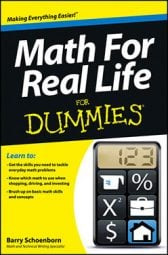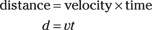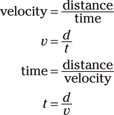##### Math For Real Life For DummiesUsing real-life math can simplify everyday situations. Math comes in handy every time you take a trip, go shopping, or do projects around the house.

## Estimating taxes and discounts when shopping

When you go shopping, you often encounter discounts and sales offers that change the price of an item. In addition, many states in the United States charge sales tax, and if you’re shopping abroad, you may face a value added tax.

To determine how much the total will be (and whether it fits into your budget or whether you have enough cash on hand to make the purchase), you need to be able to calculate the discounted price as well as determine the sales and any other taxes.

• Estimating sales tax: In the U.S., state sales taxes often end up being over 8 percent when you add in applicable local taxes. Therefore, a safe estimate is to allow for a 10 percent sales tax. A quick way to figure 10 percent of an item’s purchase price is to move the decimal point one place to the left. For example, if you buy a \$70 item at the hardware store, the tax will be approximately \$7, making the purchase price in the neighborhood of \$77.

• Estimating VAT: Many European Union and non-EU countries have a value added tax (VAT) that hovers around 20 percent. To estimate VAT, follow the same approach as you would to calculate a sales tax: Determine 10 percent by moving the decimal one place to the left. Then, double that amount. Using the \$70 purchase at the hardware store example, the VAT will be approximately \$14.

• Calculating a discount: Discounts can be for any amount: 5 percent, 10 percent, 30 percent, and so on. To do the calculations in your head, first determine what a 10 percent discount is (because that’s the easiest one to calculate) and then do a little multiplication or division to get closer to the actual discount amount.

For example, if an item is being offered at a 40 percent discount, figure 10 percent and then multiply that amount by 4. A 40 percent discount on an \$80 dress, for example, works out to be \$32 off (10 percent of \$80 is \$8, and \$8 multiplied by 4 is \$32). If the discount is 15 percent, figure the 10 percent discount, divide that number in half to determine what a 5 percent discount, and then add the two together. For the \$80 dress, you’d end up with a \$12 discount: \$8 (10 percent) + \$4 (5 percent) = \$12 (15 percent).

## Calculating area and volume for household projects

Many household projects require that you be able to calculate area or volume. Whether you’re painting your kid’s room, mulching your flowerbed for the winter, planting grass seed in the spring, or tackling any other project for which you need to find out how much of something you need to cover, knowing how to figure area and volume is a time- and money-saver.

## Calculating area

To calculate area, you simply use this formula:

area = length × width
a = lw

From there, you can get the other information you need:

• Figuring how much seed you need: Use the area formula to calculate the area you want to cover and then calculate the number of pounds of seed you need. Seed companies indicate how much coverage a pound of seed gives you. Simply divide the area you need to cover by the amount of area that 1 pound of seed covers. For example, if you want to cover an area of 1,625 square feet, and 1 pound of seed covers 600 square feet, you need about 3 pounds of seed (1,625 divided by 600 is 2.7).

• Calculating area to be painted: You can calculate the area of each wall and then add the values together, or you can determine the perimeter of the room (add the length of each wall) and multiply that value by the room’s height. A room that has a 42-foot perimeter and 8-foot ceilings has an area of 336 feet.

• Calculating how many square yards of carpeting you need: First calculate the area of the room and then convert that number from square feet to square yards by dividing the area by 9 (a square yard is 3 feet wide and 3 feet long).

## Calculating volume

When the area you want to cover has depth as well as height and width, you need to determine volume rather than area. Mulching a flowerbed is one such project: To protect your flowers, you need to spread a few inches of mulch over the whole bed.

To calculate volume, you use this formula:

volume = length × width × height
v = lwh

With that info, you can then do additional calculations to figure out how many bags of mulch to buy.

Say, for example, that you want to cover 6.68 cubic feet with mulch (that’s the volume you need for a small bed, mulching to a depth of 3 inches). Simply divide the volume you need by the number of cubic feet in a bag of mulch. If each bag holds 3 cubic feet, you need 2.23 bags. So buy 3 to be safe.

## Using your body to estimate length

Many real-life math problems don’t require exact solutions or precise calculations. When you need an approximate idea of length, you have a handy-dandy “ruler” always nearby: your body. Yes, your body — and its various parts — can serve as a quick length estimator.

• From nose to finger tip: The distance between your nose and your fingertip when your arm is extended is about a yard (36 inches). If you haven’t used this method yourself, ask your mother or grandmother: It’s the traditional way of measuring cloth.

• The distance of one full stride: Called a pace, it’s about 58 inches, just short of 6 feet. The Romans used this measurement, and so can you!

These estimates are just that: estimates. A more accurate way use your body parts to estimate length is to know your own measurements: the width of your own palm, the length of your own foot, and so on. Then, wherever you are, you’ll have a pretty accurate ruler very close by.

## Calculating speed, time, and distance

If you drive a car or have ever flown in an airplane, you’ve probably noticed that time, speed, and distance are related. Here’s the basic formula for distance (d), which equals speed (called velocity in science and represented by v) multiplied by time (t):From this simple formula, you can derive these other formulas as well:By knowing any two of the components, you can use these formulas to figure out the third. If you know how far you’ve traveled and the time the journey has taken, you can calculate your average speed. If you know the distance and the average speed, you can calculate the time you’ve been driving.

This “know two to get all three” trick applies to many day-to-day math activities: buying lumber (length needed/price per board foot/total cost), buying cases of motor oil (price per can/number of cans in a case/total cost), or buying meat at the grocery store (weight of cut/price per pound/total cost).

## Calculating GPA

Nowadays, grade point averages are a big deal, especially for college-bound students and their anxious parents. GPA is easy to calculate if you understand that it’s simply an average.

To determine an average, you add up all of the values in the group and then divide that total by the number of values in the group.

The tricky part about GPAs, however, is that you’re working with letter grades rather than numbers. For that reason, before you can calculate a GPA, you have to do a little conversion work first.

Follow these steps to calculate a GPA:

1. Convert the grades to numbers, using this scale:

A 4
B 3
C 2
D 1
F 0

Say you’re taking four courses and your grades are A, A, C, and D. The numeric equivalents are 4, 4, 2, and 1.

When you’re calculating your GPA for a semester or quarter, the task is pretty easy because people don’t usually take a lot of courses. In the example, the total is 11. The task gets a bit more challenging when you’re calculating GPA for a larger number of classes.

3. Divide by the number of courses.

In this example, divide by 4 (the number of courses). The result is a number greater than 2 and less than 3. (Actually, it’s 2 with a remainder of 3, which works out to be 0.75.) Your GPA is 2.75, or about a C+.